Arduino

# Make a Connect Four Game Using Arduino and an LED MatrixMay 18, 2017 by Anav Mehta
Share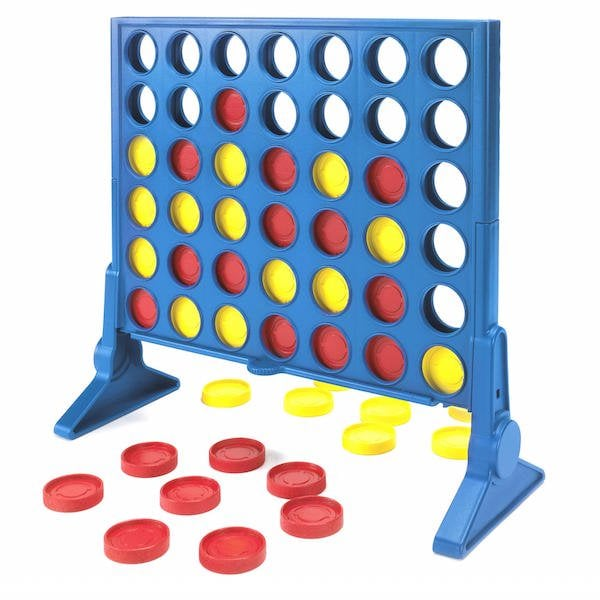Make this two-player Connect Four game using an Arduino R3 and a 7219 8×8 LED matrix.

## Hardware

 1 Arduino UNO R3 1 MAX7219 Module 1PC 1 2 axis Joystick Module 1PC

## Tools

In this project, we'll learn how to a two-player game of Connect Four using an Arduino R3 and a 7219 8x8 LED matrix.

### What Is Connect Four?

Connect Four is a two-player connection game where the players first choose a color and then take turns dropping colored discs from the top into a seven-column, six-row, vertically-suspended grid. The pieces fall straight down, occupying the next available space within the column. The objective of the game is to be the first to form a horizontal, vertical, or diagonal line of four with one's own discs. In the Arduino game, I have implemented, the player has the ability to move to any legal space in the matrix.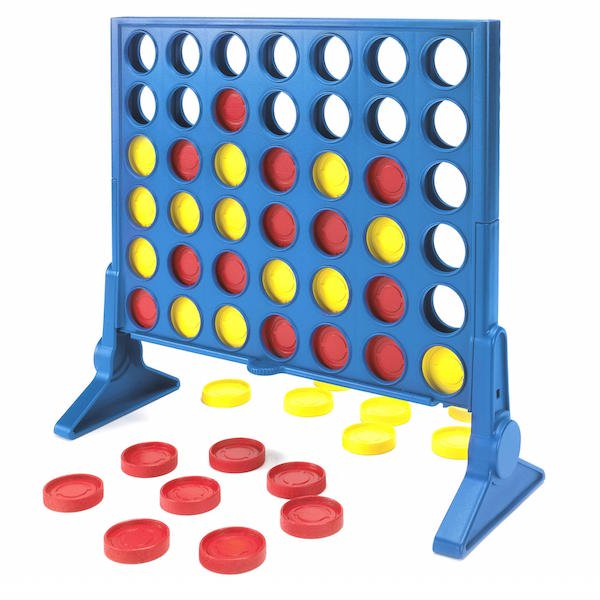The original Connect Four game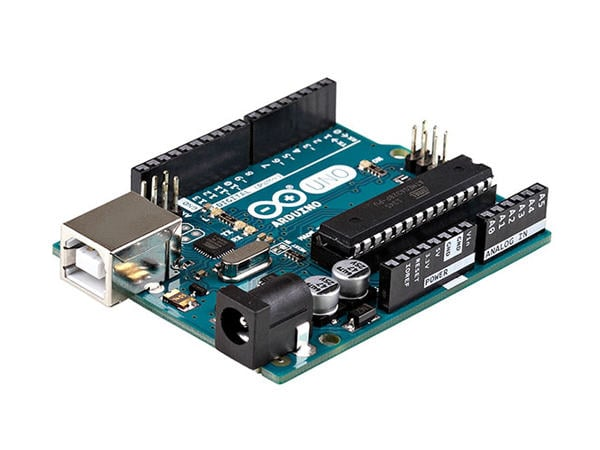Arduino Uno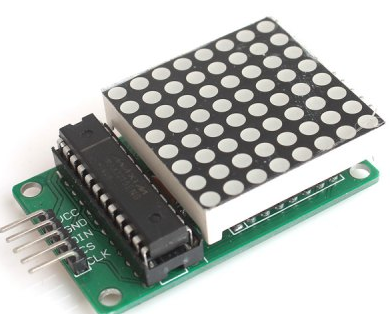MAX7219 Module (LED Matrix)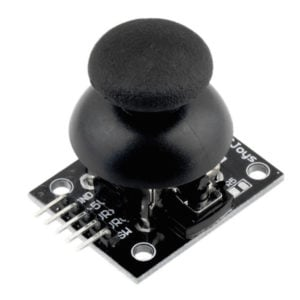2 Axis Joystick Module

### How Does the Arduino Connect Four Game Work?

Each player has a colored dot. Depending on the movement of the joystick, the values of the axis movements (X or Y) are compared and the direction of the dot on the matrix is determined. The player can move the dot up and down or left and right. Once a decision is made, the player presses down on the joystick. When it is pressed, the game switches to the next player and opponent's dot starts blinking. At each stage, the program determines if the movement of the dot is legal or not. For example, a dot cannot be moved outside the matrix or occupy the opponent's space. It also determines when the game has been won. The game is won when any player puts four dots in a line. This line can be vertical, horizontal or diagonal.

### Arduino Connect Four Game Circuit Diagram

• Connect GND on the Arduino to GND on the breadboard
• Connect 5V on the Arduino to VCC on the breadboard
• Connect D2 on the Arduino, as the digital input from the Joystick (configured as input pull-up)
• Connect pin D3 on the Arduino as the serial digital output to the LED Matrix
• Connect pin A3 on the Arduino as the analog input from the Joystick (as movement in X direction)
• Connect pin A5 on the Arduino as the analog input from the Joystick (as movement in Y direction)
• The VCC and GND inputs on the LED Matrix will be connected to the breadboard.
• We also used a power shield to provide additional power to the breadboard (This is not needed)### Arduino Code Explanation

#### Setup

The setup simply starts the Arduino with player 1 (red) and player 2 (green). Set AnalogPinX to 3, AnalogPinY to 5, and DigitalPin to 2. The LED matrix is cleared out and the initial player is set to player 1.
```void setup() {

// This is for Trinket 5V 16MHz, you can remove these three lines if you are not using a Trinket
#if defined (__AVR_ATtiny85__)
if (F_CPU == 16000000) clock_prescale_set(clock_div_1);
#endif
// End of trinket special code
delayMicroseconds(10);
rcol = 0;
gcol = 0;
bcol = 0;
rcol[player_1] = 255;
gcol[player_1] = 0;
bcol[player_1] = 0;
rcol[player_2] = 0;
gcol[player_2] = 255;
bcol[player_2] = 0;
pinMode(digitalInputPin,   INPUT_PULLUP);
currentPlayer = player_1;
Serial.begin(9600);
pixels.begin(); // This initializes the NeoPixel library.
resetgame();

}

```

#### Movement

The movement of the dots is checked on two things. First, whether the place is not already occupied (and is compared against the existing matrix). Second, whether the dot is in the matrix. If both conditions are true, then the current dot will be updated and the current location of the player will be updated as well.
```bool occupied (int x, int y, char * frame) {
int i = map(x,y);
//int val = (int) frame [(y - 1) * 8 + x - 1];
int val = (int) frame [i];
if (val != player_1 && val != player_2) {
Serial.println ("Target location is empty");
return false;
}
Serial.println ("Target location is occupied!");
return true;
}

/* move cursor up in frame */
void moveup (char * frame) {
if (csr_y_p < 8 && !occupied (csr_x_p, csr_y_p + 1, frame)) {
Serial.println ("UP!");
csr_y_c = csr_y_p + 1;
removeDot(csr_x_p, csr_y_p, frame);
placeDot(csr_x_p, csr_y_c, frame, currentPlayer);
printpos();
//delay (1000);
}
}
/* move cursor down in frame */
void movedown (char * frame) {
if (csr_y_p > 1 && !occupied (csr_x_p, csr_y_p - 1, frame)) {
Serial.println ("DOWN!");
csr_y_c = csr_y_p - 1;
removeDot(csr_x_p, csr_y_p, frame);
placeDot(csr_x_p, csr_y_c, frame, currentPlayer);
printpos();
}
}
/* move cursor left in frame */
void moveleft (char * frame) {
if (csr_x_p > 1 && !occupied (csr_x_p - 1, csr_y_p, frame)) {
Serial.println ("LEFT!");
csr_x_c = csr_x_p - 1;
removeDot(csr_x_p, csr_y_p,frame);
placeDot(csr_x_c, csr_y_p, frame, currentPlayer);
printpos();
}
}
/* move cursor right in frame */
void moveright (char * frame) {
if (csr_x_p < 8 && !occupied (csr_x_p + 1, csr_y_p, frame)) {
Serial.println ("RIGHT!");
csr_x_c = csr_x_p + 1;
removeDot(csr_x_p, csr_y_p, frame);
placeDot(csr_x_c, csr_y_p, frame, currentPlayer);
printpos();
}
}

```

#### Game Over

When a player connects four dots in a row (horizontal, vertical, or diagonal) the game is declared as over and that player wins.

```/* check if player win game by row */
bool row_win (int player, char * frame) {
for (int i = 1; i <= SIDE - K + 1; i++) {
for (int j = 1; j <= SIDE; j++) {
if (getplayer(i, j, frame) == player) {
bool win = true;
for (int k = 1; k < K; k++) {
if (getplayer(i + k, j, frame) != player) {
win = false;
break;
}
}
if (win == true) {
return true;
}
} else {
continue;
}
}
}
return false;
}
/* check if player win game by column */
bool col_win (int player, char * frame) {
for (int i = 1; i <= SIDE; i++) {
for (int j = 1; j <= SIDE - K + 1; j++) {
if (getplayer (i, j, frame) == player) {
bool win = true;
for (int k = 1; k < K; k++) {
if (getplayer(i, j + k, frame) != player) {
win = false;
break;
}
}
if (win == true) {
return true;
}
} else {
continue;
}
}
}
return false;
}
/* check if player win game by diagonal */
bool dia_win (int player, char * frame) {
// check left/up to right/down win
for (int i = 1; i <= SIDE - K + 1; i++) {
for (int j = SIDE; j >= SIDE - K; j--) {
if (getplayer (i, j, frame) == player) {
bool win = true;
for (int k = 1; k < K; k++) {
if (getplayer (i + k, j - k, frame) != player) {
win = false;
break;
}
}
if (win == true) {
return true;
}
} else {
continue;
}
}
}
// check left/down to right/up win
for (int i = 1; i <= SIDE - K + 1; i++) {
for (int j = 1; j <= SIDE - K + 1; j++) {
if (getplayer (i, j, frame) == player) {
bool win = true;
for (int k = 1; k < K; k++) {
if (getplayer (i + k, j + k, frame) != player) {
win = false;
break;
}
}
if (win == true) {
return true;
}
} else {
continue;
}
}
}
return false;
}

/* check if game is over */
int gameover () {
if (row_win (player_1, frameBuffer) ||
col_win (player_1, frameBuffer) ||
dia_win (player_1, frameBuffer)) {
for(int i=0;i<5;i++){
turnOff();
delay(500);
sendFrame(frameBuffer);
}
return player_1;
}
if (row_win (player_2, frameBuffer) ||
col_win (player_2, frameBuffer) ||
dia_win (player_2, frameBuffer)) {
for(int i=0;i<5;i++){
turnOff();
delay(500);
sendFrame(frameBuffer);
}
return player_2;
}
return INPROGRESS;
}```

### Future Work

I'm currently working on code to make a single player version with an automated opponent. You can find the full Connect Four Code on my Github.